# Test: Regular Expressions & Finite Automata- 2

## 20 Questions MCQ Test GATE Computer Science Engineering(CSE) 2023 Mock Test Series | Test: Regular Expressions & Finite Automata- 2

Description
Attempt Test: Regular Expressions & Finite Automata- 2 | 20 questions in 60 minutes | Mock test for Computer Science Engineering (CSE) preparation | Free important questions MCQ to study GATE Computer Science Engineering(CSE) 2023 Mock Test Series for Computer Science Engineering (CSE) Exam | Download free PDF with solutions
QUESTION: 1

### Which one of the following is TRUE?

Solution:

(A) L = {a n b n |n >= 0} is not regular because there does not exists a finite automaton that can derive this grammar. Intuitively, finite automaton has finite memory, hence it can’t track number of as. It is a standard CFL though.
(B) L = {a n b n |n is prime} is again not regular because there is no way to remember/check if current n is prime or not. Hence, no finite automaton exists to derive this grammar, thus it is not regular.
(C) L = {w|w has 3k+1 bs} is a regular language because k is a fixed constant and we can easily emulate L as a ∗ ba ∗ .....ba ∗ such that there are exactly 3k + 1 bs and a ∗ s surrounding each b in the grammar.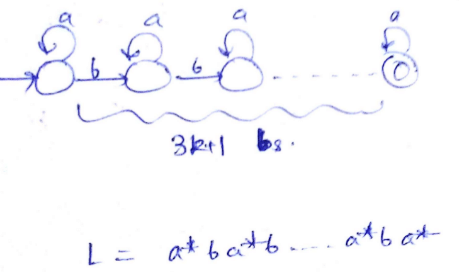(D) L = {ww| w ∈ Σ ∗ } is again not a regular grammar, infact it is not even a CFG. There is no way to remember and derive double word using finite automaton. Hence, correct answer would be (C).

QUESTION: 2

Solution:
QUESTION: 3

### Which of the regular expressions given below represent the following DFA?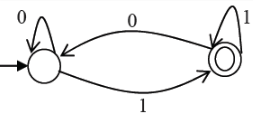I) 0*1(1+00*1)* II) 0*1*1+11*0*1 III) (0+1)*1

Solution:

I) 0*1(1+00*1)*
II) 0*1*1+11*0*1
III) (0+1)*1

(I) and (III) represent DFA.

(II) doesn't represent as the DFA accepts strings like 11011, but the regular expression doesn't accept.

QUESTION: 4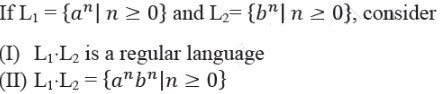Q. Which one of the following is CORRECT?

Solution:

L1.L2 is also regular since regular languages are closed under concatenation.
But, L1.L2 is not { anbn | n ≥ 0 }, because both the variable is independent in both languages.
It should have been L1.L2 = { ambn | m ≥ 0, n ≥ 0 }

QUESTION: 5

Let L1 = {w ∈ {0,1} | w has at least as many occurrences of (110)’s as (011)’s}.
Let L2 = { ∈ {0,1} | w has at least as many occurrences of (000)’s as (111)’s}.

Q. Which one of the following is TRUE?

Solution:

L1 is regular let us consider the string 011011011011 In this string, number of occurrences of 011 are 4 but when we see here 110 is also occurred and the number of occurrence of 110 is 3. Note that if i add a 0 at the last of string we can have same number of occurrences of 011 and 110 so this string is accepted. We can say if the string is ending with 011 so by appending a 0 we can make 110 also. Now string2: 110110110110 in this number of occurrences of 110 is 4 and 011 is 3 which already satisfy the condition So we can observe here that whenever 110 will be there string will be accepted So with this idea we can build an automata for this. Therefore, it is regular.

QUESTION: 6

The length of the shortest string NOT in the language (over Σ = {a, b}) of the following regular expression is ______________.

a*b*(ba)*a*

Solution:

The string "bab" is the shortest string not acceptable by regular expression.

QUESTION: 7

If s is a string over (0 + 1)* then let n0(s) denote the number of 0’s in s and n1(s) the number of 1’s in s. Which one of the following languages is not regular?

Solution:

Languages in option (A) And (D) are finite so both the options are eliminated. For option A: There are finite no. of 3 digit prime numbers. There exists a FA for every finite set. Hence FA is possible. For option D: Possible remainders for 7 is 0 to 6, and for 5 its 0 to 4. Using 35 states, FA can be made. For option B: We can have 6 states (including 1 reject state) state 1: difference is 0 state 2: difference is 1 (more 1s) state 3: difference is 1 (more 0s) state 4: difference is 2 (more 1s) state 5: difference is 2 (more 0s) state 6: reject state for difference >= 3 Suppose the string is 000101 Scan 0 -> state 3 Scan 0 -> state 5 Scan 0 -> reject (since diff. is 3 now) Similarly if we try for string: 010100, this will be accepted.

QUESTION: 8

Consider the regular language L = (111 + 11111)*. The minimum number of states in any DFA accepting this languages is:

Solution:

It is given that language L = (111 + 11111)*
Strings , that belongs in the language are
L = {null , 111 , 11111, 111111 , 11111111 , 111111111 , 1111111111 , ……. form string length 8 , (number of 1’s) , now we can can generate any length of string from length 3 and 5 (i.e. length 8 ,length 9, length 10 , length 11 ,…etc)}
L = {null , 111 , 11111, 111111 , 11111111 , 111111111* }
Strings in length , that belongs in the language
L = {0 ,3, 5, 6, 8, 9, 10, 11, …}
So, there are 5 states that are final states and 4 states that are non-final states
Therefore total number of states are 9 states .

QUESTION: 9

Consider the machine M: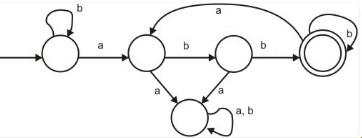Q. The language recognized by M is :

Solution:

Here w ∈ {a, b}* means w can be any string from the set of {a, b}* and {a, b}* is set of all strings composed of a and b (any string of a and b that you can think of) like null, a, b, aaa, abbaaa, bbbbb, aaaaa, aaaabbbbaabbababab etc.

These type of questions are frequently asked in GATE, where it is asked to choose best fit language among the options. To slove the question like this, there is a better way, we try to eliminate wrong options by choosing testing strings intelligently until we are left with one right option.As given in question, let’s we try to eliminate option (A), it recognizes only those string (composed of a and b) in which every a in w is followed by exactly two b’s , so if we take string abbb(three b’s), then it is accepted by machine , so this options is wrong. Now we try to eliminate option (C), it recognizes only those strings(composed of a and b) in which w contains the substring ‘abb’, so if we take string abbaa (has substring abb), then it is not accepted by machine, so this options is also wrong. Now we try to eliminate option (D), it recognizes only those string(composed of a and b) in which w does not contains ‘aa’ as a substring , so if we take string abbaba(‘aa’ not as a substring), then it is not accepted by machine ,so this options is also wrong. Only option with which we are left, is option (b) in which every a in w is followed by at least two b’ ,is correct.So answer is option (B).

QUESTION: 10

Let Nf and Np denote the classes of languages accepted by non-deterministic finite automata and non-deterministic push-down automata, respectively. Let Df and Dp denote the classes of languages accepted by deterministic finite automata and deterministic push-down automata, respectively. Which one of the following is TRUE?

Solution:

Deterministic pushdown automata can recognize all deterministic context-free languages while nondeterministic ones can recognize all context-free languages. Mainly the former are used in parser design.

Deterministic context-free languages (DCFL) are a proper subset of context-free languages. Non-deterministic finite automata and Deterministic finite automata, both accept same set of languages as NFAs can be translated to equivalent DFAs using the subset construction algorithm.

QUESTION: 11

The following diagram represents a finite state machine which takes as input a binary number from the least significant bit.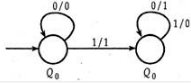Q. Which one of the following is TRUE?

Solution:

The given finite state machine takes a binary number from LSB as input.
The given FSM remains unchanged till first ‘1’ . After that it takes 1’s complement of rest of the input string.
We assume the input string to be ‘110010’ . Thus, according to the FSM, output is ‘001110’ .
2’s complement of ‘110010’ = 1’s complement of ‘110010’ + 1 = 001101 + 1 = 001110 Thus, the FSM computes 2’s complement of the input string.

Hence, option (B) is correct.

Please comment below if you find anything wrong in the above post.

QUESTION: 12

The following finite state machine accepts all those binary strings in which the number of 1's and 0's are respectively.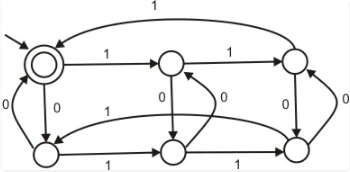Solution:

Option (B) is eliminated because string 100 contains odd number of 1s and even number of 0s but is not accepted by the DFA. Option(C) is eliminated because string 011 contains even number of 1s and odd number of 0s but is not accepted by the DFA. Option (D) is eliminated because string 11000 has number of 1s divisible by 2 and number of 0s divisible by 3 but still not accepted by the DFA. Option (A) accepts all strings with number of 1s divisible by 3 and number of 0s divisible by 2.

Extra note: In any case where (no of 1s) MOD N= some integer k and (no of 0s) MOD M= some integer q the number of states in the DFA will be equal to N*M. (The product could be taken for all input alphabets.) E.g.: if we say no. of ones is even and no. of 0s is odd (we check if (no. of 1s) MOD 2=0 and (no. of 0s) MOD 2=0) so no. of states in the DFA=2*2=4. Hence option (B) and (C) can directly be eliminated as the DFA has 6 states and we can look only at the remaining two options.

QUESTION: 13

The regular expression 0*(10*)* denotes the same set as

Solution:

Given regular expression is 0*(10*)*
A: (1*0)*1* All strings that can be generated from given regular expression can also be generated from this.
B: 0 + (0 + 10)* and C: (0 + 1)* 10(0 + 1)* We can generate 11 from given regular expression which is not possible with B and C
C: (0 + 1)* 10(0 + 1)* Not possible as we can produce {epsilon} from the given Regular Expression but not from C

QUESTION: 14

Consider the following deterministic finite state automaton M.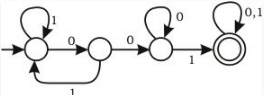Let S denote the set of seven bit binary strings in which the first, the fourth, and the last bits are 1. The number of strings in S that are accepted by M is

Solution:

Given a language of 7 bit strings where 1st, 4th and 7th bits are 1. The following are 7 strings of language that can be accepted by DFA. 1001001 1001011 1001101 1001111 1101001 1111001 1011001

QUESTION: 15

Consider the NFA M shown below.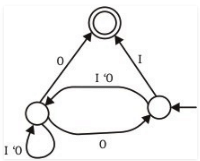Q. Let the language accepted by M be L. Let L1 be the language accepted by the NFA M1, obtained by changing the accepting state of M to a non-accepting state and by changing the non-accepting state of M to accepting states. Which of the following statements is true ?

Solution:

In case of a Deterministic Finite Automata (DFA) when we change the accepting states into non-accepting states and non-accepting states into accepting states, the new DFA obtained accepts the complement of the language accepted by the initial DFA. It is because we have one single movement for a particular input alphabet from one state so the strings accepted by the transformed DFA will be all those which are not accepted by the actual DFA.

But it is not the case with the NFA’s (Non-Deterministic Finite Automata). In case of NFA we need to have a check on the language accepted by the NFA. The NFA obtained by changing the accepting states to non-accepting states and non-accepting states to accepting states is as follows:-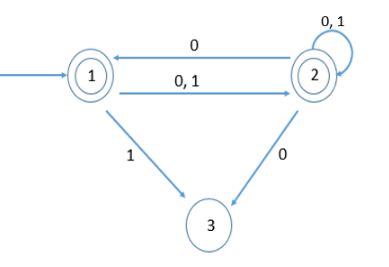Here we can see that as i. The initial state is an accepting state hence null string is always accepted by the NFA. ii. There is a movement from state 1 to state 2 on both {0, 1} input alphabets and further any number of 1’s and 0’s or even none in the string lets the string be at an accepting state(state 2).

Hence the language accepted by the NFA can be any string with any combination of 0’s and 1’s including a null string i.e. {null, 0, 1, 00, 01, 10, 11,……………..} so L1= {0, 1}*.

QUESTION: 16

The Finite state machine described by the following state diagram with A as starting state, where an arc label is x / y and x stands for 1-bit input and y stands for 2- bit output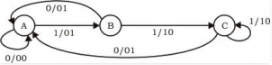Solution:

We assume the input string to be 1101.
1. (A, 1) --> (B, 01) Here, previous input bit + present input bit = 0 + 1 = 01 = output
2. (B, 1) --> (C, 10) Here, previous input bit + present input bit = 1 + 1 = 10 = output
3. (C, 0) --> (A, 01) Here, previous input bit + present input bit = 1 + 0 = 01 = output
4. (A, 1) --> (B, 01) Here, previous input bit + present input bit = 0 + 1 = 01 = output

Thus, option (A) is correct.

Please comment below if you find anything wrong in the above post.

QUESTION: 17

The smallest finite automation which accepts the language {x | length of x is divisible by 3} has :

Solution:

Input set = {1}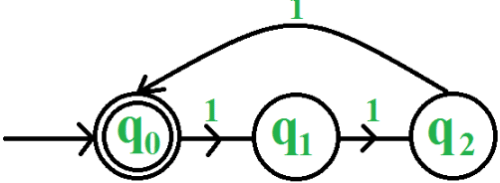Thus, we require 3 states.

So, B is the correct choice.

Please comment below if you find anything wrong in the above post.

QUESTION: 18

Consider the following two statements: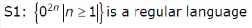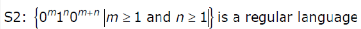Solution:

We can easily build a DFA for S1. All we need to check is whether input string has even number of 0's. Therefore S1 is regular. We can't make a DFA for S2. For S2, we need a stack. Therefore S2 is not regular.

QUESTION: 19

Given an arbitary non-deterministic finite automaton (NFA) with N states, the maximum number of states in an equivalent minimized DFA is at least

Solution:
QUESTION: 20

Consider a DFA over ∑ = {a, b} accepting all strings which have number of a’s divisible by 6 and number of b’s divisible by 8. What is the minimum number of states that the DFA will have?

Solution:

We construct a DFA for strings divisible by 6. It requires minimum 6 states as length of string mod 6 = 0, 1, 2, 3, 4, 5
We construct a DFA for strings divisible by 8. It requires minimum 8 states as length of string mod 8 = 0, 1, 2, 3, 4, 5, 6, 7
If first DFA is minimum and second DFA is also minimum then after merging both DFAs resultant DFA will also be minimum. Such DFA is called as compound automata.
So, minimum states in the resultant DFA = 6 * 8 = 48

Thus, option (D) is the answer.

Please comment below if you find anything wrong in the above post.Use Code STAYHOME200 and get INR 200 additional OFF Use Coupon Code